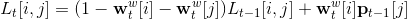# Inplace matrix modification

Is it possible to do the following matrix inplace modification using python for loop without breaking the autograd?Yes it is. This should work:

``````L = Variable(torch.Tensor(i_size, j_size))
# it's important to not specify requires_grad=True
# it makes sense - you don't need grad w.r.t. original L content,
# because it will be overwritten.
for i in range(i_size):
for j in range(j_size):
L[i, j] = # compute the value here
``````

But beware, it might be very very slow! Not only because you’ll be looping over elements in Python, but also because it will involve a lot of autograd ops to compute this, and there’s a constant overhead associated with each one. It’s not a huge problem if you’re doing relatively expensive computation like matrix multiplication or convolution, but for simple ops it can be more expensive than the computation alone.

In the vast majority of cases it is possible to rewrite the equations so that you don’t have to compute the individual elements in the loop, but you can use only a few matrix-matrix operations that achieve the same thing, but will compute the results in C using highly optimized routines. For examples you can look at how @fmassa rewrote the loss function in another thread.

1 Like

Thanks for the explanation, it is very useful!
But I have another question, In my case, L is updated at each timestep, and the output at each timestep is calculated based on the new L and weights W.

``````loss = 0
L = Variable(torch.Tensor(i_size, j_size))
W = Parameter(torch.Tensor(20, 20)) #fake size
for t in range(time_step):
for i in range(i_size):
for j in range(j_size):
L[i, j] = func(L[i,j]) # a function of wrt old L
out = get_output(L, W)  #output computed from L and weights W
loss  += loss_func(out, label[t])

``````

In this case, can I still get gradient of loss wrt the weights W using autograd? It seems that L is overwritten at each timestep.

Yes, of course, it will work. Autograd has built in checks for in-place modifications, so if it doesn’t raise an error, it will work. Otherwise `.clone()` might help you. One important thing is that you shouldn’t reuse the same `L` Variable indefinitely - its history is going to get longer and longer. You can probably reuse it for a single training sequence, but then you should do sth like `repackage_hidden` from the language modelling example, to allow the graph to get freed.

Tahnks！ That’s exactly what I want to know.